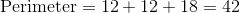# SSAT Upper Level Math : How to find the perimeter of an acute / obtuse triangle

## Example Questions

← Previous 1

### Example Question #1 : How To Find The Perimeter Of An Acute / Obtuse Triangle

The side lengths of a triangle are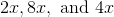. Find the perimeter of the triangle.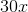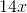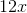Explanation:

To find the perimeter of a triangle, add up its side lengths.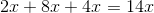### Example Question #1 : How To Find The Perimeter Of An Acute / Obtuse Triangle

The side lengths of a triangle, in inches, are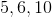. Find the perimeter of this triangle.Explanation:

Find the perimeter of a triangle by adding up all its sides.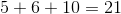### Example Question #3 : How To Find The Perimeter Of An Acute / Obtuse Triangle

A triangle has side lengths, and. What is the perimeter of this triangle?Explanation:

To find the perimeter of a triangle, add up all the side lengths.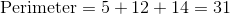### Example Question #4 : How To Find The Perimeter Of An Acute / Obtuse Triangle

In terms of, find the perimeter of a triangle with side lengths of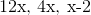.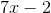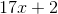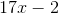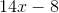Explanation:

To find the perimeter of a triangle, add up all of its sides.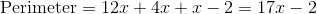### Example Question #5 : How To Find The Perimeter Of An Acute / Obtuse Triangle

In terms of, find the perimeter of a triangle with side lengths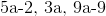.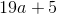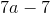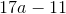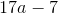Explanation:

To find the perimeter of a triangle, add up all of its sides.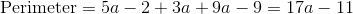### Example Question #6 : How To Find The Perimeter Of An Acute / Obtuse Triangle

In terms of, find the perimeter of a triangle with side lengths of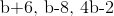.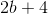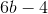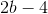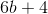Explanation:

To find the perimeter of a triangle, add up all of its sides.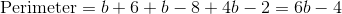### Example Question #7 : How To Find The Perimeter Of An Acute / Obtuse Triangle

In terms of, find the perimeter of a triangle with side lengths of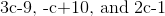.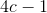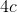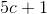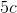Explanation:

To find the perimeter of a triangle, add up all of its sides.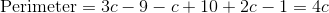### Example Question #8 : How To Find The Perimeter Of An Acute / Obtuse Triangle

Find the perimeter of a triangle with side lengths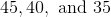.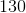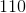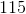Explanation:

To find the perimeter of a triangle, add up all of its sides.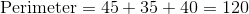### Example Question #9 : How To Find The Perimeter Of An Acute / Obtuse Triangle

Bill has a triangular garden that he needs to fence. The garden has side lengths of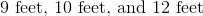. In feet, how much fencing will Bill need?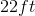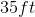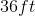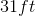Explanation:

To find how much fencing Bill needs, you will need to find the perimeter of the triangle. The perimeter of a triangle is found by adding up all the sides together.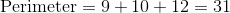### Example Question #10 : How To Find The Perimeter Of An Acute / Obtuse Triangle

Find the perimeter of a triangle with side lengths of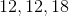.